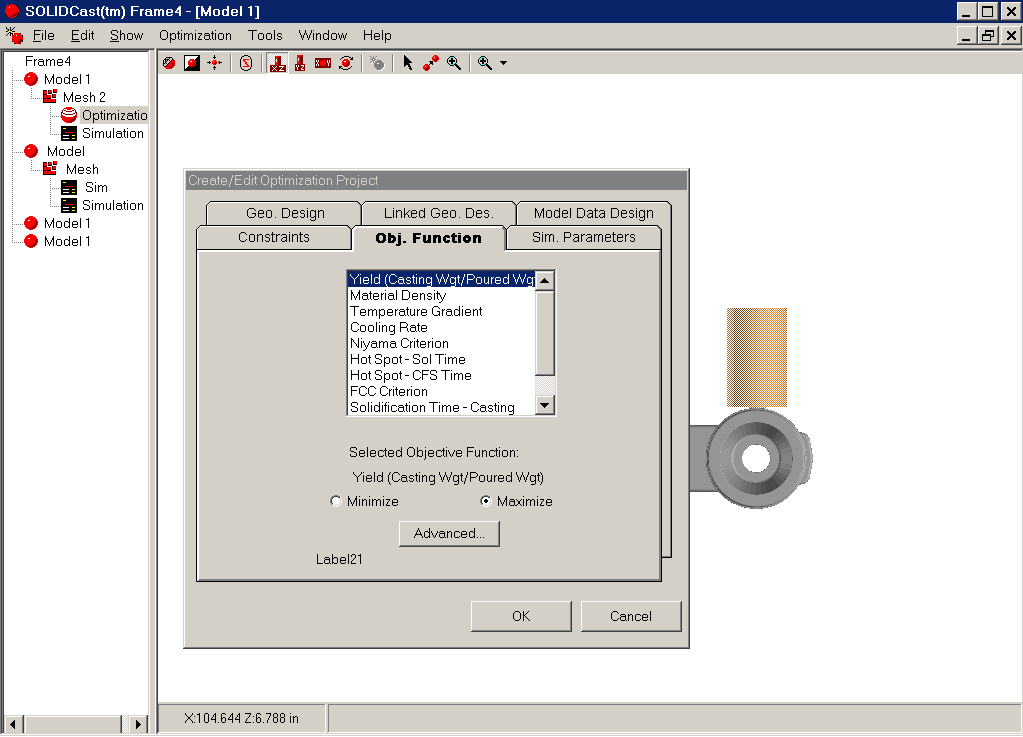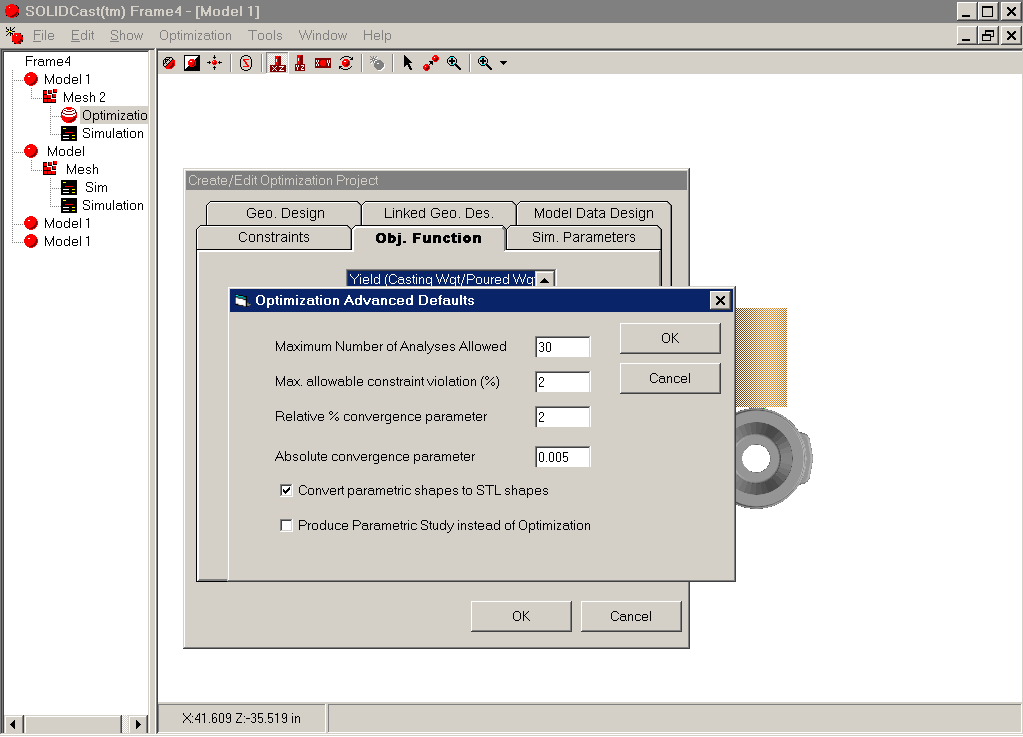# UNIT 10: Advanced Optimization Parameters

When creating or editing an Optimization Project, and you are on the Obj. Function Tab, one of the options is to set up Advanced Optimization Parameters. This is done by clicking on the Advanced… button, as shown below:This will bring up a window with the Optimization Advanced Defaults, as shown on the next page. These settings are described on the following pages.MAXIMUM NUMBER OF ANALYSES ALLOWED - The parameter tells OPTICast how many simulations it may run, as a maximum, in searching for an optimum solution. As a very rough rule of thumb, the number of simulations required is the square of the number of Design Variables. If you have 4 DV's, the system may take around 16 simulations to find an optimum. It is a good idea to set this parameter a little higher, so a good setting with 4 DV's might be 25-30.

Remember that if you are allowing Vertical Scale and Horizontal Scale to vary for a riser, this makes 2 DV's for that riser.

MAX. ALLOWABLE CONSTRAINT VIOLATION (%) - OPTICast allows constraints to be violated by a small amount. This parameter gives OPTICast a "band" to work in to evaluate whether a design is feasible, i.e., whether it violates a constraint or not.

A good working number for this would typically be in the range of 1.5% to 2.0%.

Too small a percentage may make it difficult for OPTICast to find a solution.

RELATIVE % CONVERGENCE PARAMETER - When searching for an optimum condition, OPTICast looks at the Objective Function value on each subsequent simulation. If subsequent values are within this percentage, then OPTICast decides that it has found an optimum condition.

A good working number for this would typically be in the range of 1.5% to 2.0%.

If this value is too small, OPTICast may have trouble finding a true optimum.

ABSOLUTE CONVERGENCE PARAMETER - This is another parameter which OPTICast uses to look at subsequent values of the Objective Function and determine whether an optimum has been reached. Instead of a percentage, this parameter looks at the absolute difference in values from one simulation to the next.

A suggested starting value might be 0.005.

CONVERT PARAMETRIC SHAPES TO STL SHAPES - In SOLIDCast, model shapes can be parametric (such as a cylinder) or STL shapes (as imported from CAD in STL format). When stretching shapes vertically and horizontally, there may be situations where a parametric shape may not stretch correctly.

For example, a cylinder is always perfectly round. If a horizontal cylinder is scaled by one factor vertically and another factor horizontally, the result may not be correct since the cylinder will refuse to be elliptical in shape.

This parameter converts ALL shapes in the model to STL shapes, which can be scaled in ANY direction. We recommend turning "ON" this parameter.

PRODUCE PARAMETRIC STUDY INSTEAD OF OPTIMIZATION - There are times when you might like to evaluate how much effect a single Design Variable has on a given Objective Function (simulation output). Instead of running an optimization, you can select a Single-Variable Parametric Study. You must have specified only ONE Design Variable (Remember: Vertical and Horizontal Scale Factors are EACH DV's).

By selecting a Parametric Study, the system will ask how many study points to include. For example, six study points will cause OPTICast to run six simulations with the DV varying in even increments. You can then see how this single variable affects the output.